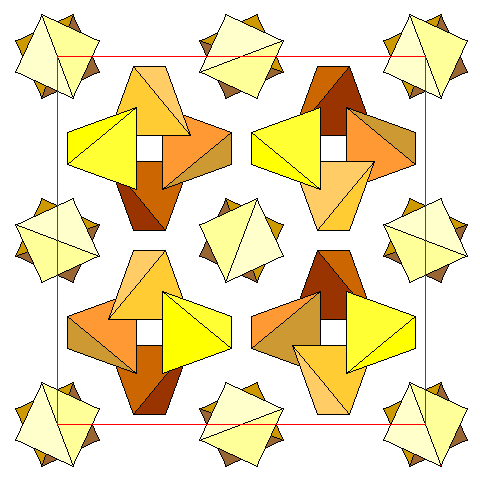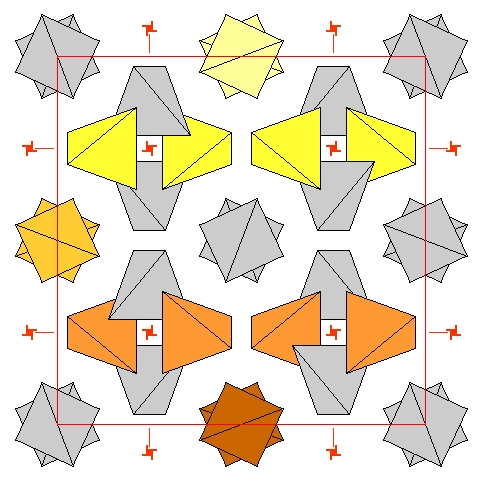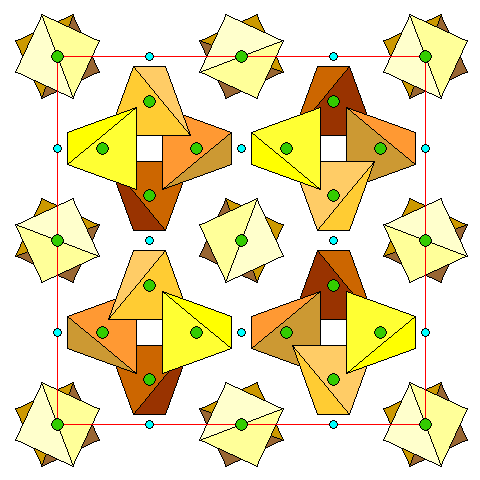# Garnet Structure

Steven Dutch, Professor Emeritus, Natural and Applied Sciences, Universityof Wisconsin - Green Bay

Garnet is one of the most common nesosilicates but it has a complex structure. We can visualize the structure one cation at a time

The general formula of garnet is A3B2Si3O12,where A is a divalent cation (generally Mg, Fe, Mn or Ca) and B is trivalent (Usually Al, sometimes Fe+3, Ti, or Cr). Since Ca is much larger inradius than the other divalent cations, there are are two series of garnets: one with calcium and one without.

## The Silica Tetrahedra

In all diagrams below, we define the visible face of the unit cell to be at altitude +1, and the opposite face at zero. Altitudes of coordination polyhedra are always given in terms of their central cation.Silica tetrahedra in the garnet unit cell. Tetrahedra are darker with increasing distance below the plane of the diagram. With the visible face of the unit cell  defined as +1, and the opposite face as zero, the tetrahedra around the four-fold screw axes have their central Si atoms at  altitudes 0,  1/4, 1/2, and 3/4, and the pairs of tetrahedra at 3/8 and 7/8.In the diagram above, it looks like there are two distinct sets of tetrahedra. In reality there are not.  This diagram shows the rows of tetrahedra  related by  the leftmost vertical screw axis. There are rows of tetrahedra parallel to all the unit cell edges, all arranged around fourfold screw axes. The apparently isolated pairs of tetrahedra are really the same as those in the four-fold groups, merely viewed along their length instead of perpendicular to it.

## The CationsDivalent cations are in green, trivalent in blue. As we can see from the end-on rows, each row of tetrahedra lies along a twofold symmetry axis. Divalent cations lie on the twofold axes midway between tetrahedra. For example, consider the topmost two yellow tetrahedra, which lie on a horizontal two-fold axis. Their corresponding divalent ion is halfway between them, superposed on the orange tetrahedron. The divalent cations shown superposed on the yellow tetrahedra are at altitude 5/4 and are above the unit cell. The corresponding tetrahedra are not shown but they lie directly above the tetrahedra at altitude 1/4. The trivalent cations are between tetrahedra at different elevations.

## The Trivalent CationsThe trivalent cations are easiest to visualize because they are octahedrally coordinated with oxygen.  Since the symmetry is isometric, this view is simultaneously a top view and a side view. We can see that there is one set of trivalent ions at altitudes 0, 1/2 and +1, and another at 1/4 and 3/4. The light blue octahedra are at altitude 1/2, the dark blue octahedra are at 1/4.

## The Divalent Cations

The coordination of the divalent cations is harder to see. They are in eight-fold coordination with oxygen, but the bond lengths and angles are not uniform and the resulting coordination polyhedra are not simple. The simplest visualization is to regard the polyhedra as cubes with warped faces.This diagram shows the distorted cube coordination polyhedra for the divalent cations.

Created 8 December 2001, Last Update